Altair® Monarch®

# Grouping Columns

Group allows you to classify or consolidate rows that belong together according to some common value within the row. When you consolidate the records, you can also select column values to aggregate.

For example, records like these, that show Customers and Items and Quantities: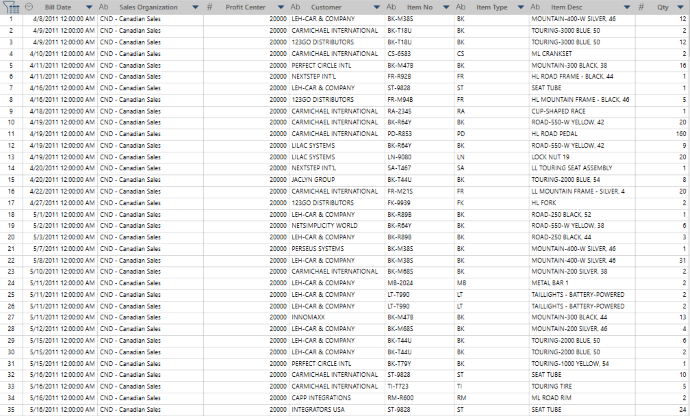Can be grouped by Customer and Item, to find out the total item quantities bought by each customer: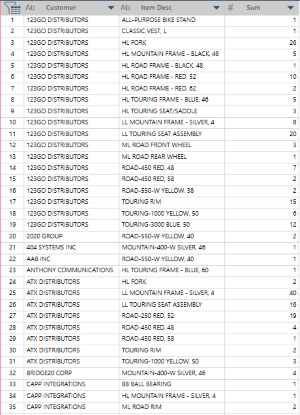Note that You can only group columns from the Prepare Window.

#### Steps:

1. In the Prepare window, select the table you want to group.

2. Select Transform on the Monarch Data Prep Studio Toolbar.

A dialog box allows you to select a transformation.

1. Select Group By to display the Group By dialog:1. Set Grouping options:

• Enter the name of the resulting table

• Click the Case Sensitive box if you want to differentiate values based on case. For instance, CD and cd are considered two distinct values if case sensitivity is on, and will result in two groups for this value.

1. Select the columns to group on by selecting the key icon beside a column on the Value Columns section of the dialog box: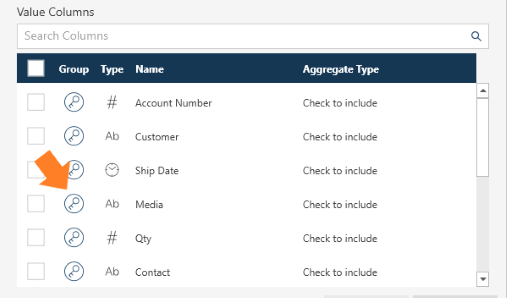In the example above, we are selecting Media as a group by column. Once selected the column is moved to the Group Columns section.

1. Select the columns to include in the transform.

To select the specific columns to use as values, check the box beside a column.

To select all columns check the box on the header row.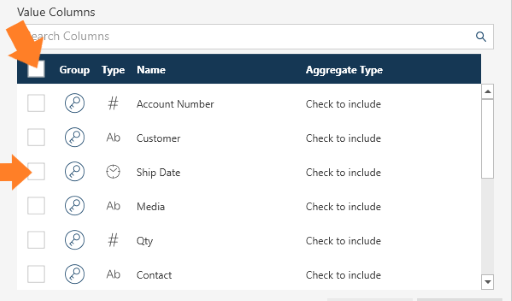1. If applicable, select the aggregate operation to use.

To do so,  select the operation from the drop-down beside a value column you have selected to include  in the grouping: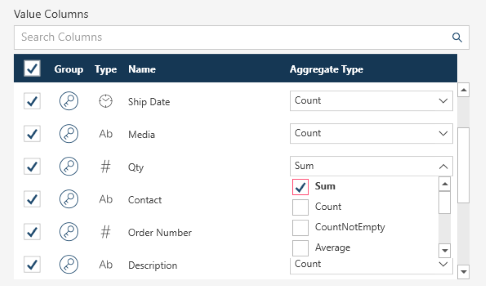In the example above, we are including Qty in the grouping and have selected Sum as an aggregation.

Notes:

• Monarch Data Prep Studio uses a default name for the aggregation. You can change this using the New column name column.

• Different operations are available for specific data types:

Operation

Valid for

Description

Sum

number

Computes the sum of all item values in the group.

Example:

 TOTAL AMOUNT VALUE Amount 1 10,000.00 Amount 2 20,000.00 Amount 3 30,000.00 Amount 4 40,000.00 Amount 5 50,000.00

Sum (Total Amount)  = 150,000.00

Average

number

Computes the  average (sum of all item values divided by the number of items)  of all the values in the group.

Example:

 TOTAL AMOUNT VALUE Amount 1 10,000.00 Amount 2 20,000.00 Amount 3 30,000.00 Amount 4 40,000.00 Amount 5 50,000.00

Average (Total Amount)  = 150,000.00 /5 = 30,000

CountNotEmpty

number

Counts the number of non-empty items in the group:

Example:

 TOTAL AMOUNT VALUE Amount 1 10,000.00 Amount 2 Amount 3 30,000.00 Amount 4 Amount 5 50,000.00

CountNotEmpty (Total Amount)  = 50,000.00

Maximum

number

Returns  the maximum value among all item values in the  group.

Example:

 TOTAL AMOUNT VALUE Amount 1 10,000.00 Amount 2 20,000.00 Amount 3 30,000.00 Amount 4 40,000.00 Amount 5 50,000.00

Maximum (Total Amount)  = 50,000.00

Median

number

Returns  the median value among all item values in the  group.

Example:

 TOTAL AMOUNT VALUE Amount 1 10,000.00 Amount 2 20,000.00 Amount 3 30,000.00 Amount 4 40,000.00 Amount 5 50,000.00

Median (Total Amount)  = 30,000.00

Minimum

number

Returns  the minimum  value among all item values in the  group.

Example:

 TOTAL AMOUNT VALUE Amount 1 10,000.00 Amount 2 20,000.00 Amount 3 30,000.00 Amount 4 40,000.00 Amount 5 50,000.00

Minimum (Total Amount)  = 10,000.00

Count

number, date, text

Counts the number of items in the group.

Example:

 TOTAL AMOUNT VALUE Amount 1 10,000.00 Amount 2 20,000.00 Amount 3 30,000.00 Amount 4 40,000.00 Amount 5 50,000.00

Count (Total Amount)  = 5

First

number, date, text

Displays the first value on the table.

Example:

 TOTAL AMOUNT VALUE Amount 1 40,000.00 Amount 2 20,000.00 Amount 3 30,000.00 Amount 4 40,000.00 Amount 5 50,000.00

First (Total Amount)  = 40,000.00

Last

Displays the last value on the table.

Example:

 TOTAL AMOUNT VALUE Amount 1 40,000.00 Amount 2 20,000.00 Amount 3 30,000.00 Amount 4 40,000.00 Amount 5 10,000.00

Last (Total Amount)  = 10,000.00

1. Select OK.

Monarch Data Prep Studio applies the grouping and  creates a new table.